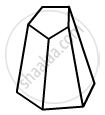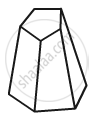Advertisement Remove all ads

# Verify Euler'S Formula for the Following Polyhedron: - Mathematics

Answer in Brief

Verify Euler's formula for the following polyhedron:Advertisement Remove all ads

#### Solution

In the given polyhedron:

Edges E=15

Faces F=7

Vertices V=10Now, putting these values in Euler's formula:

LHS: F+V

= 7+10

= 17

LHS: E + 2

= 15 + 2

= 17

LHS = RHS

Hence, the Euler's formula is satisfied.

Is there an error in this question or solution?
Advertisement Remove all ads

#### APPEARS IN

RD Sharma Class 8 Maths
Chapter 19 Visualising Shapes
Exercise 19.1 | Q 6.1 | Page 9
Advertisement Remove all ads
Advertisement Remove all ads
Share
Notifications

View all notifications

Forgot password?
Course